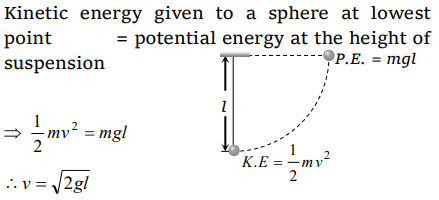## Motion in a Plane Questions and Answers Part-12

1. A mass is supported on a frictionless horizontal surface. It is attached to a string and rotates about a fixed centre at an angular velocity $\omega_{0}$ . If the length of the string and angular velocity are doubled, the tension in the string which was initially $T_{0}$ is now
a) $T_{0}$
b) $T_{0}\diagup 2$
c) $4T_{0}$
d) $8T_{0}$

Explanation:2. A particle is moving in a circle of radius R with constant speed v, if radius is double then its centripetal force to keep the same speed should be
a) Doubled
b) Halved
d) Unchanged

Explanation:3. A stone ties to the end of a string 1m long is whirled in a horizontal circle with a constant speed. If the stone makes 22 revolution in 44m seconds, what is the magnitude and direction of acceleration of the stone
a) $\frac{\pi^{2}}{4}ms^{-2}$  and direction along the radius towards the centre
b) $\pi^{2}ms^{-2}$  and direction along the radius away from the centre
c) $\pi^{2}ms^{-2}$  and direction along the radius towards the centre
d) $\pi^{2}ms^{-2}$  and direction along the tangent to the circle

Explanation:4. A particle describes a horizontal circle in a conical funnel whose inner surface is smooth with speed of 0.5 m/s. What is the height of the plane of circle from vertex of the funnel ?
a) 0.25 cm
b) 2 cm
c) 4 cm
d) 2.5 cm

Explanation:5. What is the angular velocity of earth
a) $\frac{2\pi}{86400}rad\diagup sec$
b) $\frac{2\pi}{3600}rad\diagup sec$
c) $\frac{2\pi}{24}rad\diagup sec$
d) $\frac{2\pi}{6400}rad\diagup sec$

Explanation: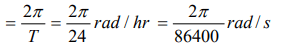6. If the length of the second's hand in a stop clock is 3 cm the angular velocity and linear velocity of the tip is

Explanation: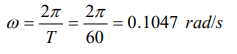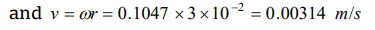7. In a circus stuntman rides a motorbike in a circular track of radius R in the vertical plane. The minimum speed at highest point of track will be
a) $\sqrt{2gR}$
b) $2gR$
c) $\sqrt{3gR}$
d) $\sqrt{gR}$

Explanation: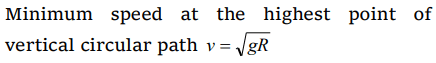8. A block of mass m at the end of a string is whirled round in a vertical circle of radius R . The critical speed of the block at the top of its swing below which the string would slacken before the block reaches the top is
a) Rg
b) $\left(Rg\right)^{2}$
c) $R\diagup g$
d) $\sqrt{Rg}$

Explanation:9. A sphere is suspended by a thread of length l . What minimum horizontal velocity has to be imparted the ball for it to reach the height of the suspension
a) gl
b) 2gl
c) $\sqrt{gl}$
d) $\sqrt{2gl}$